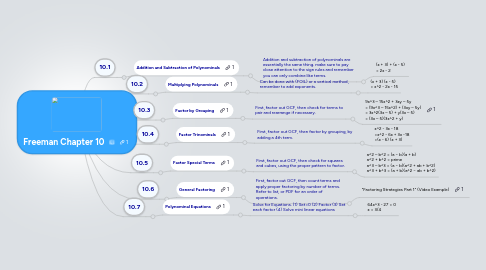# Freeman Chapter 10

Get Started. It's Free
or sign up with your email addressFreeman Chapter 10## 1. 10.2

### 1.1. Multiplying Polynominals

1.1.1. Can be done with (FOIL) or a vertical method, remember to add exponents.

1.1.1.1. (x + 3) (x - 5) = x^2 - 2x - 15

## 2. 10.3

### 2.1. Factor by Grouping

2.1.1. First, factor out GCF, then check for terms to pair and rearrange if necessary.

2.1.1.1. 9x^3 – 15x^2 + 3xy – 5y = (9x^3 – 15x^2) + (3xy – 5y) = 3x^2(3x – 5) + y(3x – 5) = (3x – 5)(3x^2 + y)

## 3. 10.4

### 3.1. Factor Trinominals

3.1.1. First, factor out GCF, then factor by grouping, by adding a 4th term.

3.1.1.1. x^2 - 3x - 18 =x^2 - 6x + 3x -18 =(x - 6) (x + 3)

## 4. 10.7

### 4.1. Polynominal Equations

4.1.1. Solve for Equations. (1) Set=0 (2) Factor (3) Set each factor (4) Solve mini linear equations

4.1.1.1. 64x^3 - 27 = 0 x = 3/4

## 5. 10.6

### 5.1. General Factoring

5.1.1. First, factor out GCF, then count terms and apply proper factoring by number of terms. Refer to list, or PDF for an order of operations.

5.1.1.1. "Factoring Strategies Part 1" (Video Example)

## 6. 10.5

### 6.1. Factor Special Terms

6.1.1. First, factor out GCF, then check for squares and cubes, using the proper pattern to factor.

6.1.1.1. a^2 – b^2 = (a − b)(a + b) a^2 + b^2 = prime a^3 – b^3 = (a − b)(a^2 + ab + b^2) a^3 + b^3 = (a + b)(a^2 − ab + b^2)

## 7. 10.1

### 7.1. Addition and Subtraction of Polynominals

7.1.1. Addition and subtraction of polynominals are essentially the same thing. make sure to pay close attention to the sign rules and remember you can only combine like terms.

7.1.1.1. (x + 3) + (x - 5) = 2x - 2